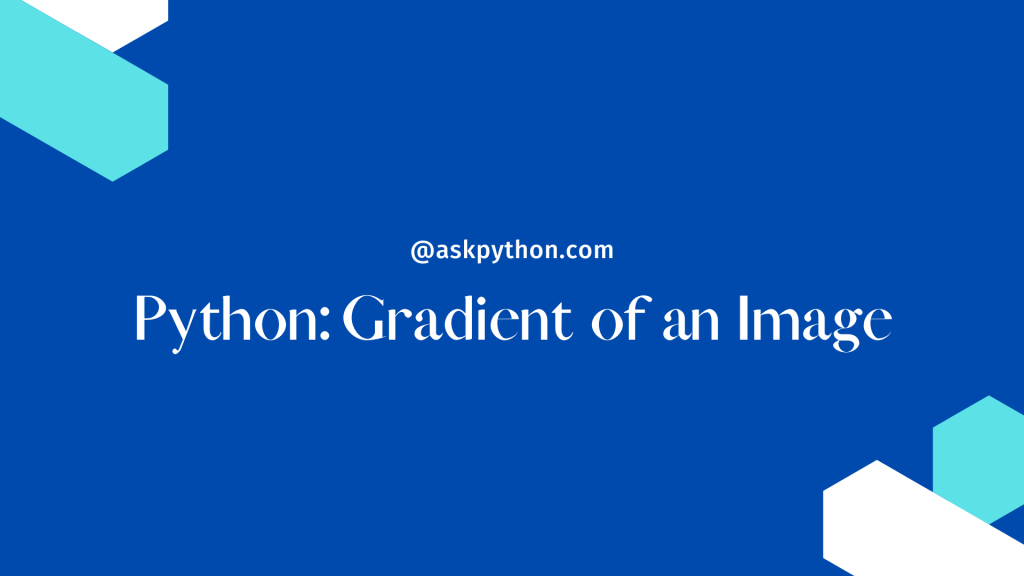# Finding the Gradient of an Image Using PythonWe will learn how to find the gradient of a picture in Python in this tutorial. After completing this course, you will be able to identify the gradient of a picture in X, Y, and both directions, as well as utilize several useful libraries.

The gradient picture is nothing more than a shift in the intensity of image colors in X, Y, or both directions.

The gradient of a picture may be determined using the image’s Sobel and Laplacian derivatives. Sobel can be employed in either the X or Y direction, or both, but Laplacian can aid in both directions.

## Importing Required Modules

First, we import our OpenCV cv2 libraries, Numpy, and Matplotlib. Following that, we used the imread() method of cv2 with two arguments to read our picture.

The first is our picture name with extension (make sure it is in the same folder as the image) and the second is scale type, which is either 0,1,-1 only in numeric form. Grayscale, color, and nochange are the three scale types.

```import cv2
import numpy as np
import matplotlib.pyplot as plot
```

## Finding the Gradient of an Image Using Python

Following that, we will use the Python Laplacian() to determine the image’s Laplacian derivatives by giving three parameters. The first is our image variable, the second is the data type CV 64F of cv2, and the third is the kernel size. 3 for ksize (make sure always use odd number)

The value of ksize increases the thickness of the edges. ksize 3 will get the greatest results. Following that, we will convert the value to an unsigned 8-bit integer of Numpy’s uint8 type.

After that, we compute the gradient of the picture in the x or y direction using Sobel() of cv2 by supplying four parameters: the first is the image source, the second is depth, the third is x derivatives, and the fourth is y derivatives in the y-direction dy.

```lap = cv2.Laplacian(image,cv2.CV_64F,ksize=3)
lap = np.uint8(np.absolute(lap))

sobelx= cv2.Sobel(image,0, dx=1,dy=0)
sobelx= np.uint8(np.absolute(sobelx))

sobely= cv2.Sobel(image,0, dx=0,dy=1)
sobely = np.uint8(np.absolute(sobely))
```

Finally, we make two lists: one for the title and one for pictures created by the laplacian, sobelx, and sobely.

After utilizing matplotlib, we plot on a screen with the imshow() function, supplying two parameters: one for the image source and one for the backdrop. yticks() and xticks() can be used with a list (which can be empty) to set the labels in the x and y directions.

```results = [lap,sobelx,sobely]
plt.figure(figsize=(10,10))
for i in range(3):
plot.title(results[i])
plot.subplot(1,3,i+1)
plot.imshow(results[i],"plasma")
plot.xticks([])
plot.yticks([])

plot.show()
```